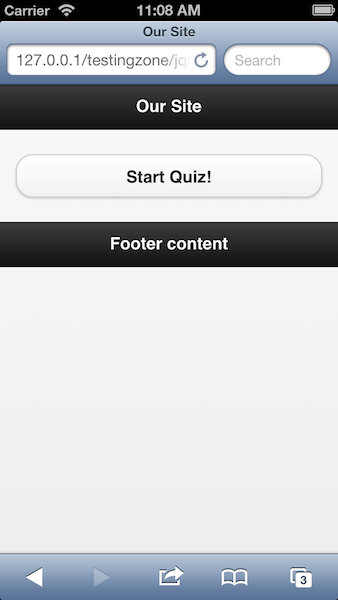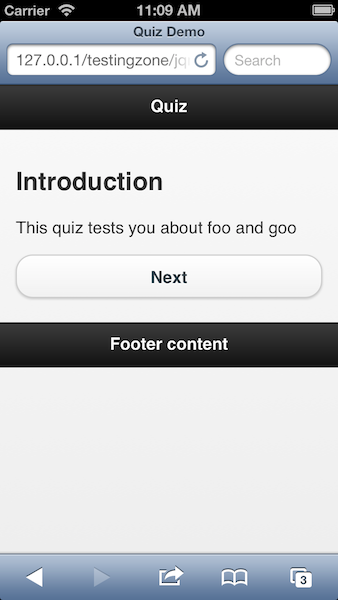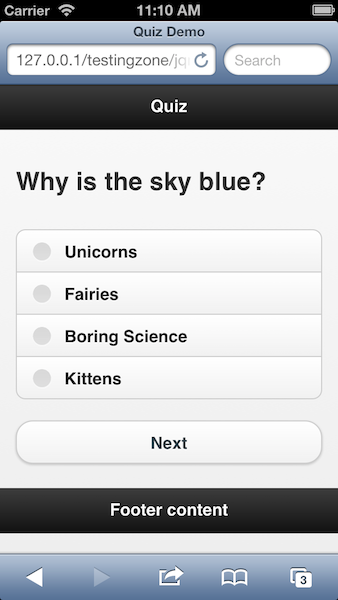A few weeks ago a reader asked if I had ever designed a quiz for jQuery Mobile. While I had not, I spent some time thinking about how a quiz could be designed as well as how a generic library could help automate it. I've built a demo I'd like to share with folks. It is definitely "First Draft" (but hey, it lints!) so feel free to tear it apart and suggest improvements.

I began by thinking how quiz data could be represented. I figured either XML or JSON. JSON has the benefit of being really easy to work with in JavaScript. XML has the benefit of being really easy to write, even for non-devs. At the end of the day though I settled on JSON. My library could be updated to handle both though. Here is an example quiz I used for my demo.

``````{
"introduction":"This quiz tests you about foo and goo",
"questions":[
{"question":"Why is the sky blue?",
"correct":2},
{"question":"Why are kittens so cute?",
"correct":3}
]
}``````

The scheme consists of an optional introduction and an array of questions. Each question has a question value (the actual text), an array of answers, and a correct index. This is 0-based but I'm thinking it may make sense to be 1-based. My design only allows for multiple choice questions with one answer, but you could also do true/false of course.

On the jQuery Mobile side, the library is used by running an execute method. The execute method takes the URL of a quiz, a DOM element to render the quiz within, and a success callback. My jQuery Mobile application uses this app.js file to handle that aspect:

``````/* global \$,document,console,quizMaster */

\$(document).on("pageshow", "#quizPage", function() {
console.log("Page show");
//initialize the quiz
quizMaster.execute("q1.json", ".quizdisplay", function(result) {
console.log("SUCESS CB");
console.dir(result);
});
});
}); ``````

I whipped up a quick jQuery Mobile application with two pages. The first simply links over to the quiz page.Once you load the quiz page, the code you see above runs the library. Here is how the quiz displays the introduction:And this is the first question:Ok, now let's look at the library.

``````/* global \$,window */
var quizMaster = (function () {
var name;
var data;
var displayDom;
var successCbAlias;

function nextHandler(e) {
e.preventDefault();

var status = getUserStatus();

//if we aren't on the intro, then we need to ensure you picked something
if(status.question >= 0) {
if(checked.length === 0) {
return;
} else {
}
}
status.question++;
storeUserStatus(status);
displayQuiz(successCbAlias);
}

function displayQuiz(successCb) {

//We copy this out so our event can use it later. This feels wrong
successCbAlias = successCb;
var current = getQuiz();
var html;

if(current.state === "introduction") {
html = "<h2>Introduction</h2><p>" + current.introduction + "</p>" + nextButton();
displayDom.html(html).trigger('create');
} else if(current.state === "inprogress") {

html = "<h2>" + current.question.question + "</h2><form><div data-role='fieldcontain'><fieldset data-role='controlgroup'>";
}
html += "</fieldset></div></form>" + nextButton();
displayDom.html(html).trigger('create');
} else if(current.state === "complete") {
html = "<h2>Complete!</h2><p>The quiz is now complete. You got "+current.correct+" correct out of "+data.questions.length+".</p>";
displayDom.html(html).trigger('create');
removeUserStatus();
successCb(current);
}

//Remove previous if there...
//Note - used click since folks will be demoing in the browser, use touchend instead
displayDom.off("click", ".quizMasterNext", nextHandler);
//Then restore it
displayDom.on("click", ".quizMasterNext", nextHandler);

}

function getKey() {
return "quizMaster_"+name;
}

function getQuestion(x) {
return data.questions[x];
}

function getQuiz() {
//Were we taking the quiz already?
var status = getUserStatus();
if(!status) {
storeUserStatus(status);
}
//If a quiz doesn't have an intro, just go right to the question
if(status.question === -1 && !data.introduction) {
status.question = 0;
storeUserStatus(status);
}

var result = {
currentQuestionNumber:status.question
};

if(status.question == -1) {
result.state = "introduction";
result.introduction = data.introduction;
} else if(status.question < data.questions.length) {
result.state = "inprogress";
result.question = getQuestion(status.question);
} else {
result.state = "complete";
result.correct = 0;
for(var i=0; i < data.questions.length; i++) {
result.correct++;
}
}
}
return result;
}

function getUserStatus() {
var existing = window.sessionStorage.getItem(getKey());
if(existing) {
return JSON.parse(existing);
} else {
return null;
}
}

function nextButton() {
return "<a href='' class='quizMasterNext' data-role='button'>Next</a>";
}

function removeUserStatus(s) {
window.sessionStorage.removeItem(getKey());
}

function storeUserStatus(s) {
window.sessionStorage.setItem(getKey(), JSON.stringify(s));
}

return {
execute: function( url, dom, cb ) {
//We cache the ajax load so we can do it only once

\$.get(url, function(res, code) {
//Possibly do validation here to ensure basic stuff is present
name = url;
data = res;
displayDom = \$(dom);
//console.dir(res);
displayQuiz(cb);
});

} else {
displayQuiz(cb);
}
}
};
}());``````

There's a lot here and I'll try to explain it bit by bit. The end of the code is the public API which - for now - has one method, execute. Note how we detect if the quiz is already loaded. This way we can cache the JSON and not load it after we've fetched it once in the request.

displayQuiz is the main handler for rendering the quiz. It begins (ignore the copy statement) by calling getQuiz. getQuiz handles interfacing with the quiz data as well as the user data. I'm using sessionStorage to remember where you are in the quiz. This is useful if you leave the quiz before finishing it. getQuiz also does some intelligent handling of state. So for example, if there isn't an introduction it ensures you go right into the first question. It also recognizes when you're done and checks your work.

Back in displayQuiz we use the result of getQuiz to render one of three states - the introduction, the quiz itself, or the completion. By the way, the success callback is used to allow your calling code to record the results to your server via AJAX, or do anything really.

All in all this was fun to write, but as I said, feels very much like a first draft. Want to try it yourself? Hit the demo link below.